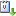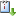Usted está aquí: Multi-clustered solutions for a forced pendulum equation

# Multi-clustered solutions for a forced pendulum equation

Ponente: Dora Salazar
Tipo de Evento: Investigación
Cuándo 17/03/2016 de 10:00 a 11:00 Salón 2vCaliCal

We consider the singularly perturbed forced pendulum equation
\begin{equation*}
\varepsilon^2 u_{\varepsilon}^{\prime\prime}+\sin (u_{\varepsilon})=\varepsilon^2\alpha(t) u_{\varepsilon}+\varepsilon^2\beta(t) u_{\varepsilon}^{\prime}\qquad \text{in } (-L,L),
\end{equation*}
where $$\alpha,\beta\in C^2([-L,L],\mathbb{R})$$ and  $$u_{\varepsilon}$$ represents the angle of the pendulum.

We shall present some recent results concerning the asymptotic behaviour of high energy solutions of this equation as the parameter $$\varepsilon$$ approaches zero.

We shall also  prove the existence of a family of solutions having a prescribed  asymptotic profile and exhibiting a highly rotatory behaviour alternated with a highly oscillatory behaviour in some open subsets of the domain. The proof of these results relies on a combination of the Nehari finite dimensional reduction with the topological degree theory.

This is a joint work with Salomé Martínez (Universidad de Chile).